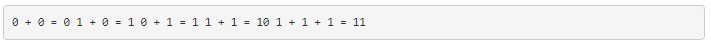## The Rules of Binary Addition

Adding binary numbers is a very simple task, and very similar to the longhand addition of decimal numbers. As with decimal numbers, you start by adding the bits (digits) one column, or place weight, at a time, from right to left. Unlike decimal addition, there is little to memorize in the way of rules for the addition of binary bits:Just as with decimal addition, when the sum in one column is a two-bit (two-digit) number, the least significant figure is written as part of the total sum and the most significant figure is “carried” to the next left column. Consider the following examples:The addition problem on the left did not require any bits to be carried since the sum of bits in each column was either 1 or 0, not 10 or 11. In the other two problems, there definitely were bits to be carried, but the process of addition is still quite simple.

## Binary Addition is the Foundation of Digital Computers

As we’ll see later, there are ways that electronic circuits can be built to perform this very task of addition, by representing each bit of each binary number as a voltage signal (either “high,” for a 1; or “low” for a 0). This is the very foundation of all the arithmetic which modern digital computers perform.